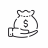# Simple ATR Trailing Stop Not Working

T

##### Member
Hey guys, I'm baffled that I cannot get this simple ATR trailing stop attempt working correctly. I just want to setup a basic ATR trailing stop that stays on the intended side of a trade (as opposed to the built-in indicator that flips sides depending on price action). For some reason, though, if you plot the following code the plot does not obey the Max or Min functions and will decrease (if max used) or increase (if min used) with price action. Anyone know what I'm doing wrong?

Code:
``````input ATRPeriod = 5;
input ATRFactor = 3.5;

def loss = ATRFactor * atr(atrperiod);
def traillong = close - loss;
def trailshort = close + loss;

plot atrtraillong = Max(traillong, traillong);
plot atrtrailshort = Min(trailshort, trailshort);``````

M

#### mashume

##### Well-known member
VIP
Hey guys, I'm baffled that I cannot get this simple ATR trailing stop attempt working correctly. I just want to setup a basic ATR trailing stop that stays on the intended side of a trade (as opposed to the built-in indicator that flips sides depending on price action). For some reason, though, if you plot the following code the plot does not obey the Max or Min functions and will decrease (if max used) or increase (if min used) with price action. Anyone know what I'm doing wrong?

Code:
``````input ATRPeriod = 5;
input ATRFactor = 3.5;

def loss = ATRFactor * atr(atrperiod);
def traillong = close - loss;
def trailshort = close + loss;

plot atrtraillong = Max(traillong, traillong);
plot atrtrailshort = Min(trailshort, trailshort);``````

Does this do something like what you want?

Code:
``````input ATRPeriod = 5;
input ATRFactor = 3.5;

def loss = ATRFactor * atr(atrperiod);
def traillong = close - loss;
def trailshort = close + loss;
def bar = barnumber();

def atrtraillong = if bar >= ATRPeriod then max(atrtraillong, traillong) else traillong;
def atrtrailshort =  if bar >= ATRPeriod then min(atrtrailshort, trailshort) else trailshort;

plot atr_long = atrtraillong;
plot atr_short = atrtrailshort;``````

-mashume

T

##### Member
@mashume Unfortunately not.. that just looks to plot horizontal lines very far from the price action.

The behavior I'm looking for is to plot a line that trails the highest close price - ATR * multiplier, and a second plot that trails the lowest close + ATR * multiplier, just like the built-in ATR Trailing Stop indicator does. However, the built-in indicator swaps sides of the price when price closes above/below the trailing stop. I just want 2 lines that stay on their side: one above the price, one below.

I still can't figure out why my code isn't working, and I greatly appreciate any help you or anyone can give!

X

#### XeoNoX

##### Member
Not sure what you are trying to do, but here you go with labels to show you that the values are correct. The Highest 4 day price minus the ATR multiplied by 1.

Code:
``````#####

declare upper;

def ATRLength = 4;
input averagetype = AverageType.SIMPLE;
def BasePeriod = AggregationPeriod.FOUR_DAYS;
input showlabel = yes;
input showBubble = yes;
input ShiftBubble = 5;
Input Multiplied_by = 1;
def n1 = ShiftBubble + 1;

def ATR1 = MovingAverage (averagetype, TrueRange(high(period = BasePeriod), close(period = BasePeriod), low(period = BasePeriod)), ATRLength);

def Todays_High = Highest(high(period = BasePeriod), 1);
def Todays_Low = Lowest(low(period = BasePeriod), 1);

plot Todays_High_Multiplied = (todays_High - atr1) *(Multiplied_by) ;
plot Todays_Low_Multiplied = (todays_Low -atr1) * (Multiplied_by);

Todays_High_Multiplied.SetDefaultColor(Color.GRAY);
Todays_Low_Multiplied.SetDefaultColor(Color.GRAY);
Todays_High_Multiplied.SetPaintingStrategy(PaintingStrategy.HORIZONTAL);
Todays_Low_Multiplied.SetPaintingStrategy(PaintingStrategy.HORIZONTAL);

def cond = showBubble and IsNaN(close[ShiftBubble]) and !IsNaN(close[n1]) ;
AddChartBubble(cond, Todays_High_Multiplied, Concat("Todays High: ", Round(Todays_High_Multiplied)), Color.MAGENTA);
AddChartBubble(cond, Todays_Low_Multiplied, Concat("Todays Low: ", Round(Todays_Low_Multiplied)), Color.MAGENTA);
AddLabel(showlabel, "4 Day ATR High: " + Round(todays_High , 2)  + " (" + atrlength + "D.Avg): ",color.DARK_ORANGE);
AddLabel(showlabel, "4 Day ATR Low: " + Round(todays_Low , 2)  + " (" + atrlength + "D.Avg): ",color.DARK_ORANGE);
AddLabel(showlabel, "ATR Range: " + Round(ATR1 , 2)  + " (" + atrlength + "D.Avg): ",color.DARK_ORANGE);``````

T

##### Member
I really appreciate the help - you gave me exactly what I asked for. I realize now that I'm a dummy and what I really wanted to accomplish is not possible without implimenting logic to say if I'm in a trade or not, since the plots have to disappear otherwise if I'm flat.

What I am trying to accomplish is just to have a trailing stop when I enter into orders, so that I can effectively capture profits. I want the magnitude of that trailing stop to = ATR * multiplier.

Actually, after looking at it again, @mashume made just what I need, except I need the plot to start over again each time an order is entered. I'm not even sure this is possible to use when backtesting, like I'd like, since I'm not sure if you can get it to recognize any of the Portfolio functions in this context (I haven't been able to while using the below change to @mashume's code)

Code:
``````def atrtraillong = if isnan(entryprice()) then double.NaN else if bar >= ATRPeriod then max(atrtraillong, traillong) else traillong;
def atrtrailshort =  if isnan(entryprice()) then double.NaN else if  bar >= ATRPeriod then min(atrtrailshort, trailshort) else trailshort;``````

I've no doubt you guys are far more clever than me and can figure out a way, though!

X

#### XeoNoX

##### Member
cant get your portifolio number from what i know of. manual entry is needed.

•M

#### mashume

##### Well-known member
VIP
@TraderKevin I wrote some code somewhere around here that looked at whether you were in a trade for trying to plot ToS mobile style lines. You look at
Code:
``if isnan(entryprice) and !isnan(entryprice) then IN_TRADE else NOT_IN_TRADE``
but that's pseudo-code not real code to put in your script.

-mashumeSimple visual indicator for a line over another line Questions 8